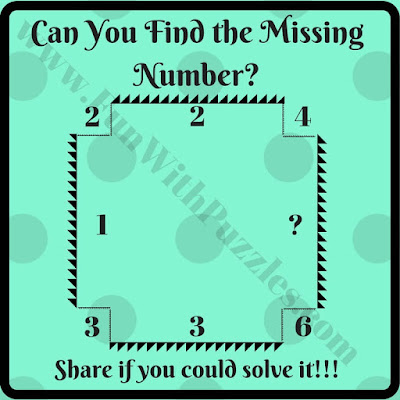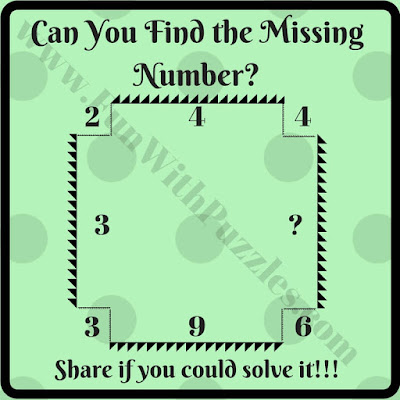Mathematics is a very fun and interesting subject. There are many Maths Brain Teasers that are very fun to solve. These Maths Brain Teasers not only are fun to solve but also help us to boost our mathematical and analytical abilities. Here is a set of Math Brain Teasers that can be used by school-going students to enhance and test their mathematical abilities.

## How to Solve Maths Brain Teasers

In these Maths Brain Teasers, Numbers are given in and around rectangles. These numbers are related to each other with some mathematical sequence/pattern or with some mathematical formulas which one has to find out and then solve for one of the missing numbers in this rectangular picture.

Answers to these Fun Maths Brain Teasers are given at the end. Please post your answers with an explanation in the comment section of this post.1. An easy math brain teaser for students2. A quick math brain teaser for students3. A simple math brain teaser for students4. A mind-bending math brain teaser for students5. A tough math brain teaser for students